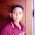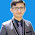## Monday, 22 May 2017

Bijoy Ekushe Is the most popular software for typing Bangla sentences. It is very easy to use. You will find all the words very easily. This software supports only Windows XP, Windows 7 and Windows Vista. You can use it on Windows 32 bit or Windows 64 bit. For Change Language you can Press on Keyboard Ctrl + Alt + V  or Ctrl + Alt + B. Almost 95 % of people use Bijoy Ekushe software for type Bangla Word.

How to Install:
2. Then double click the software.
3. One new window will come Click Yes to continue.
4. Software will be installing and click close.
5. Restart your computer and enjoy Bijoy Ekushe.

Keyboard Details:
Q =  ঙ                                         Shift + Q = ং
W =  য                                        Shift + W = য়
E =  ড                                         Shift + E = ঢ
R =  প                                         Shift + R = ফ
T =  ট                                         Shift + T = ঠ
Y =  চ                                         Shift + Y = ছ
U =  জ                                        Shift + U = ঝ
I =  হ                                          Shift + I =  ঞ
O =  গ                                         Shift + O = ঘ
P =  ড়                                         Shift + P = ঢ়
A = ‍ ‍ ‍ৃ                                           Shift + A =  র্
S =   ‍ু                                           Shift + S = ‍ ‍ূ
D =  ি                                          Shift + D =     ী
F =  া                                          Shift + F =  অ
G =  ্                                           Shift + G =  ।
H =  ব                                         Shift + H = ভ
J =  ক                                         Shift + J =  খ
K =  ত                                        Shift + K = থ
L =  দ                                         Shift + L = ধ
Z =  ্র (র ফলা)                          Shift + Z = ্য (য ফলা)
X =  ও                                         Shift + X = ৗ
C =   ে                                         Shift + C =   ৈ
V =  র                                         Shift + V =  ল
B =  ন                                         Shift + B =  ণ
N =  স                                         Shift + N =  ষ
M =  ম                                        Shift + M = শ
J + G + (Shift + N) = ক্ষ
(Shift + I ) + G + U = ঞ্জ
U + G + ( Shift + I ) = জ্ঞ
Q + G + O = ঙ্গ

1.2.Really good post like it thank you sir

1.Welcome sir

3.it's vary easy to use.

1.yes. Thanks for stay with us.

4.please visit my website for more technology tips and tricks: https://www.galaxyoftechnologies.com/

1.5.